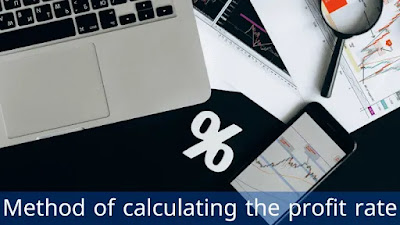# Method of calculating the profit rate

### Profit and stock market

Profit affects not only investors and creditors, but also the stock market, as public companies listed on the stock market announce their financial results every three months in the quarterly reports, and this happens during the earnings season, and companies also expect future profits, and the season affects Profits greatly affect the performance of the stock market. If the profits are higher than expectations, this will lead to an increase in the value of the company's shares. If the profits are below expectations, it will lead to a decrease in the value of the company's shares. During this article, the method of calculating the profit percentage will be identified.Method of calculating the profit rate

### The difference between profit and profitability

Before getting to know the method of calculating the profit ratio, the difference between profit and profitability must be known. Profit is an absolute “non-relative” number that is determined by the value of income or revenue, which exceeds the costs or expenses incurred by the company. Profit is calculated by subtracting total expenses from total income and it appears in The company’s income statement. As for profitability, it is closely related to profit, but with one main difference, which is that profit is an absolute value, while profitability is a relative value. Profitability is the measure used to determine the extent of the company’s profit in relation to the size of the business. It is also a measure of efficiency and ultimately the success or failure of the company. Profitability can also be defined as the ability of firms to produce a return on investment based on their resources compared to alternative investment.

### Method of calculating the profit rate

The method of calculating the profit ratio, which is also called, the profit margin ratio, the return on sales ratio, or the gross profit ratio, which is the profitability ratio that measures the amount of net income earned with each amount of money from sales, resulting from comparing the net income and net sales of the company, in other words you Gross profit ratio or profit margin ratio by showing the percentage of sales remaining after all expenses have been paid by the company. Investors and creditors also use this ratio to measure the effectiveness of the company in converting sales into net income. Investors want to ensure that profits are high enough to distribute profits, while Creditors want to make sure that the company has enough profits to repay the loans, the rest of the external users want to know if the company is operating efficiently, and a very low profit ratio indicates that expenses are too high and management needs to budget and reduce expenses.

To complement the method of calculating the profit ratio, the profit ratio or profit margin ratio measures the percentage of sales that consist of net income, in other words, it measures the amount of profits that are produced at a certain stage of sales, and this ratio indirectly measures how the company manages its expenses in relation to its net Sales, and since generating additional revenue is more difficult than reducing expenses, so managers generally resort to reducing the spending budget to improve the profit ratio, and the following example will illustrate the method of calculating the profit ratio.

The net sales of a company amounted to \$1,000,000 and the net income was \$100,000. In this case, the profit percentage or the profit margin ratio is \$100,000 net income ÷ \$1,000,000 net sales = 10%.